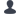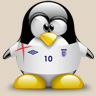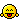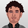FM Scout is the only community you can talk Football Manager in real time. Here are 10 reasons to join!

# Ball Control,Shooting & Set Pieces Staff?

Started on 6 April 2010 by cudi
Latest Reply on 8 April 2010 by bill777
• POSTS9
• VIEWS26416cudi
hey i was just wondering what do i need to look for when it comes to ball control, shooting & set pieces training? Like what are the attributes i should look for? also does anyone know of any good coaches, but that are cheap?iRoN420
Not my work but I can't remember where I got it from. I have had this in a notepad since fm2007. It still pretty much holds true as far as maxing out a coaches stars in a given area.

* Strength - Fitness 18+
* Aerobic - Fitness 18+
* Goalkeeping - Coaching Goalkeepers 18+
* Tactics - Tactical 18+

* Defending - Defending 20 and Tactical 10+ or
* Defending - Defending 19 and Tactical 14+ or
* Defending - Defending 18 and Tactical 18+

* Ball Control - Technical 20 and Mental 10+ or
* Ball Control - Technical 19 and Mental 14+ or
* Ball Control - Technical 18 and Mental 18+

* Attacking - Attacking 20 and Tactical 10+ or
* Attacking - Attacking 19 and Tactical 14+ or
* Attacking - Attacking 18 and Tactical 18+

* Shooting - Technical 20 and Attacking 10+ or
* Shooting - Technical 19 and Attacking 14+ or
* Shooting - Technical 18 and Attacking 18+

* Set Pieces - Tech 20, Ment/Attacking = 30+ Combined (eg 20+10, 15+15) or
* Set Pieces - Tech 19, Ment/Attacking = 33+ Combined (eg 20+13, 17+16) or
* Set Pieces - Tech 18, Ment/Attacking = 36+ Combined (eg 20+16, 18+18) or
* Set Pieces - Tech 17, Ment/Attacking = 39+ Combined (eg 20+19, 20+20)schnopsnosn
don't forget level of disciplinebill777
* Strength:
Fitness * 9 + ddm * 2
>= 270 = 5 stars
>= 240 = 4.5 stars
>= 210 = 4 stars
>= 180 = 3.5 stars
>= 150 = 3 stars
>= 120 = 2.5 stars
>= 90 = 2 stars
>= 60 = 1.5 stars
>= 30 = 1 star
>= 0 = 0.5 stars

* Aerobic:
Fitness * 9 + ddm * 2
>= 270 = 5 stars
>= 240 = 4.5 stars
>= 210 = 4 stars
>= 180 = 3.5 stars
>= 150 = 3 stars
>= 120 = 2.5 stars
>= 90 = 2 stars
>= 60 = 1.5 stars
>= 30 = 1 star
>= 0 = 0.5 stars

* Goalkeeping:
Goalkeepers * 2 + ddm
>=90 = 5 stars
>=80 = 4.5 stars
>=70 = 4 stars
>=60 = 3.5 stars
>=50 = 3 stars
>=40 = 2.5 stars
>=30 = 2 stars
>=20 = 1.5 stars
>=10 = 1 star
>=0 = 0.5 stars

* Tactics:
Tactical * 2 + ddm
>=90 = 5 stars
>=80 = 4.5 stars
>=70 = 4 stars
>=60 = 3.5 stars
>=50 = 3 stars
>=40 = 2.5 stars
>=30 = 2 stars
>=20 = 1.5 stars
>=10 = 1 star
>=0 = 0.5 stars

* Ball Control:
Technical * 6 + Mental * 3 + ddm * 2
>= 270 = 5 stars
>= 240 = 4.5 stars
>= 210 = 4 stars
>= 180 = 3.5 stars
>= 150 = 3 stars
>= 120 = 2.5 stars
>= 90 = 2 stars
>= 60 = 1.5 stars
>= 30 = 1 star
>= 0 = 0.5 stars

* Defending:
Defending * 8 + (Tactical + ddm) * 3
>=360 = 5 stars
>=320 = 4.5 stars
>=280 = 4 stars
>=240 = 3.5 stars
>=200 = 3 stars
>=160 = 2.5 stars
>=120 = 2 stars
>=80 = 1.5 stars
>=40 = 1 star
>=0 = 0.5 stars

* Attacking:
Attacking * 6 + Tactical * 3 + ddm * 2
>= 270 = 5 stars
>= 240 = 4.5 stars
>= 210 = 4 stars
>= 180 = 3.5 stars
>= 150 = 3 stars
>= 120 = 2.5 stars
>= 90 = 2 stars
>= 60 = 1.5 stars
>= 30 = 1 star
>= 0 = 0.5 stars

* Shooting:
Technical * 6 + Attacking * 3 + ddm * 2
>= 270 = 5 stars
>= 240 = 4.5 stars
>= 210 = 4 stars
>= 180 = 3.5 stars
>= 150 = 3 stars
>= 120 = 2.5 stars
>= 90 = 2 stars
>= 60 = 1.5 stars
>= 30 = 1 star
>= 0 = 0.5 stars

* Set Pieces:
(Attacking + Mental + Technical) * 3 + ddm * 2
>= 270 = 5 stars
>= 240 = 4.5 stars
>= 210 = 4 stars
>= 180 = 3.5 stars
>= 150 = 3 stars
>= 120 = 2.5 stars
>= 90 = 2 stars
>= 60 = 1.5 stars
>= 30 = 1 star
>= 0 = 0.5 stars

ddm? is easy and very important for every coach: ddm = Determination + Discipline + Motivating

P/S: thx me if u like it :p
is a exel file for who interest http://www.fileden.com/files/2007/7/23/1289074/Coach%20Stars%20for%20FM2010.xlscudi
thanks guys that realy helped alotkingdevilb
bill777
ah sorry for my ignorance but i don't get it ...
what the hell does this numbers means?
>= 270 = 5 stars
if i have 5 stars coach then what does the number means?...
270- meaning?.. what does it improves if i use this training on one for my players..
thank you...bill777
lol, dude, read it again, "270" (for example) is the result of ur calculation.
For example:

* Set Pieces:
(Attacking + Mental + Technical) * 3 + ddm * 2 = " X "

">= 270 = 5 stars"

if X >= 270 so that coach have 5 start in set pieces training

clear now?

(if u still dont get it =.=,.. how old r u? 2? or 3? )kingdevilb
ohhhh!!! now it's all makes seance lol :yes
thank you and a the same time screw you dude i am 16 years old i bet that i am better then you in math :yes
for some reason which i can't really explane, i read the equation for right to left :con lolbill777
Lol im 2o y old if u ask me ;;) , u r good in math? lol,.. kk i can see thatYou are reading "Ball Control,Shooting & Set Pieces Staff?".

###### FMS Chat–Stam
hey, just wanted to let you know that we have a fb style chat for our members. login or sign up to start chatting.